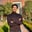Related Tags

binary tree
golang
communitycreator

# What is a binary tree in Golang?Saad Akbar

### Overview

In computer science, a binary tree is referred to as a tree-like data structure in which every node has two child nodes: left and right child nodes. These child nodes further have their own child nodes. This process continues until the leaf node is reached, which does not have child nodes. Through this process, the entire tree-like structure is produced, which is why we call this data structure a binary tree.

In this shot, we'll be implementing the binary tree through Golang.

### Example

package main

import (
"fmt"
"os"
"io"
)

type BinaryNode struct {
data  int64
left  *BinaryNode
right *BinaryNode

}

type BinaryTree struct {
root *BinaryNode
}

func (t *BinaryTree) insert(data int64) *BinaryTree {
if t.root != nil {
t.root.insert(data)

} else {
t.root = &BinaryNode{data: data, left: nil, right: nil}
}
return t
}

func (n *BinaryNode) insert(data int64) {
if n == nil {
return
} else if data >= n.data {
if n.right == nil {
n.right = &BinaryNode{data: data, left: nil, right: nil}
} else {
n.right.insert(data)
}

} else {

if n.left == nil {
n.left = &BinaryNode{data: data, left: nil, right: nil}
} else {
n.left.insert(data)
}
}
}

func print(w io.Writer, node *BinaryNode, ns int, ch rune) {
if node == nil {
return
}

for i := 0; i < ns; i++ {
fmt.Fprint(w, " ")
}
fmt.Fprintf(w, "%c:%v\n", ch, node.data)
print(w, node.left, ns+2, 'L')
print(w, node.right, ns+2, 'R')
}

func main() {
tree := &BinaryTree{}
tree.insert(50).
insert(30).
insert(-50).
insert(60).
insert(-60).
insert(20).
insert(-10).
insert(65).
insert(5).
insert(15).
insert(-5).
insert(62)
print(os.Stdout, tree.root, 0, 'M')
}
Try it out!

### Explanation

• This example shows how a binary tree is implemented in Golang. First of all, a struct is declared where each struct represents a binary node. As mentioned earlier, each binary node has a left and right child. The root node is the node where the first node of the tree is declared. All other nodes follow the root node.
• In lines 20–50, there are two insert functions. This is because the first insert function is specifically for the root node. This has a different if condition because it checks if the root node is null or not. The second insert function is specified for all the other nodes except the root. It checks if the data being entered is greater than the node value, then adds the data to the node's right or left otherwise.
• Later on, the print function is declared to print the tree. The main function creates a BinaryTree object named tree and inserts multiple values in it to populate the tree. It is then printed through the print function.

RELATED TAGS

binary tree
golang
communitycreator

CONTRIBUTORSaad Akbar
RELATED COURSES

View all Courses

Keep Exploring

Learn in-demand tech skills in half the time